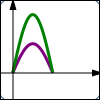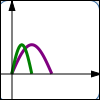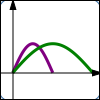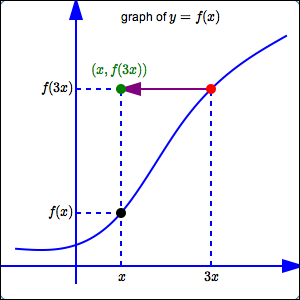﻿ Horizontal and Vertical Stretching/Shrinking

# HORIZONTAL AND VERTICAL STRETCHING/SHRINKING

• PRACTICE (online exercises and printable worksheets)$y = f(x)$ $y = 2f(x)\,$ vertical stretch;$\,y\,$-values are doubled; points get farther away from $\,x\,$-axis $y = f(x)$ $y = \frac{f(x)}{2}\,$ vertical shrink; $\,y\,$-values are halved; points get closer to $\,x\,$-axis $y = f(x)$ $y = f(2x)\,$ horizontal shrink; $\,x\,$-values are halved; points get closer to $\,y\,$-axis $y = f(x)$ $y = f(\frac x2)\,$ horizontal stretch; $\,x\,$-values are doubled; points get farther away from $\,y\,$-axis vertical stretching/shrinking changes the $y$-values of points; transformations that affect the $\,y\,$-values are intuitive horizontal stretching/shrinking changes the $x$-values of points; transformations that affect the $\,x\,$-values are counter-intuitive Vertical/Horizontal Stretching/Shrinking usually changes the shape of a graph.

The lesson Graphing Tools: Vertical and Horizontal Scaling in the Algebra II curriculum
gives a thorough discussion of horizontal and vertical stretching and shrinking.

The key concepts are repeated here.

The exercises in this lesson duplicate those in Graphing Tools: Vertical and Horizontal Scaling.
IDEAS REGARDING VERTICAL SCALING (STRETCHING/SHRINKING)
• Points on the graph of $\,y=f(x)\,$ are of the form $\,\bigl(x,f(x)\bigr)\,$.
Points on the graph of $\,y=3f(x)\,$ are of the form $\,\bigl(x,3f(x)\bigr)\,$.
Thus, the graph of $\,y=3f(x)\,$ is found by taking the graph of $\,y=f(x)\,$, and multiplying the $\,y$-values by $\,3\,$.
This moves the points farther from the $\,x$-axis, which tends to make the graph steeper.
• Points on the graph of $\,y=f(x)\,$ are of the form $\,\bigl(x,f(x)\bigr)\,$.
Points on the graph of $\,y=\frac13f(x)\,$ are of the form $\,\bigl(x,\frac13f(x)\bigr)\,$.
Thus, the graph of $\,y=\frac13f(x)\,$ is found by taking the graph of $\,y=f(x)\,$, and multiplying the $\,y$-values by $\,\frac13\,$.
This moves the points closer to the $\,x$-axis, which tends to make the graph flatter.
• Transformations involving $\,y\,$ work the way you would expect them to work—they are intuitive.
• Here is the thought process you should use when you are given the graph of $\,y=f(x)\,$
and asked about the graph of $\,y=3f(x)\,$: \begin{align} \text{original equation:} &\quad y=f(x)\cr\cr \text{new equation:} &\quad y=3f(x) \end{align} $$\begin{gather} \text{interpretation of new equation:}\cr\cr \overset{\text{the new y-values}}{\overbrace{ \strut\ \ y\ \ }} \overset{\text{are}}{\overbrace{ \strut\ \ =\ \ }} \overset{\quad\text{three times}\quad}{\overbrace{ \strut \ \ 3\ \ }} \overset{\qquad\text{the previous y-values}\quad}{\overbrace{ \strut\ \ f(x)\ \ }} \end{gather}$$
• Summary of vertical scaling:

Let $\,k \gt 1\,$.
Start with the equation $\,y=f(x)\,$.
Multiply the previous $\,y\,$-values by $\,k\,$, giving the new equation $\,y=kf(x)\,$.
The $\,y$-values are being multiplied by a number greater than $\,1\,$, so they move farther from the $\,x$-axis.
This tends to make the graph steeper, and is called a vertical stretch.

Let $\,0 \lt k \lt 1\,$.
Start with the equation $\,y=f(x)\,$.
Multiply the previous $\,y\,$-values by $\,k\,$, giving the new equation $\,y=kf(x)\,$.
The $\,y$-values are being multiplied by a number between $\,0\,$ and $\,1\,$, so they move closer to the $\,x$-axis.
This tends to make the graph flatter, and is called a vertical shrink.

In both cases, a point $\,(a,b)\,$ on the graph of $\,y=f(x)\,$ moves to a point $\,(a,k\,b)\,$ on the graph of $\,y=kf(x)\,$.
This transformation type is formally called vertical scaling (stretching/shrinking).
IDEAS REGARDING HORIZONTAL SCALING (STRETCHING/SHRINKING)
 Points on the graph of $\,y=f(x)\,$ are of the form $\,\bigl(x,f(x)\bigr)\,$. Points on the graph of $\,y=f(3x)\,$ are of the form $\,\bigl(x,f(3x)\bigr)\,$. How can we locate these desired points $\,\bigl(x,f(3x)\bigr)\,$? First, go to the point $\,\color{red}{\bigl(3x\,,\,f(3x)\bigr)}\,$ on the graph of $\,\color{red}{y=f(x)}\,$. This point has the $\,y$-value that we want, but it has the wrong $\,x$-value. The $\,x$-value of this point is $\,3x\,$, but the desired $\,x$-value is just $\,x\,$. Thus, the current $\,\color{purple}{x}$-value must be divided by $\,\color{purple}{3}\,$; the $\,\color{purple}{y}$-value remains the same. This gives the desired point $\,\color{green}{\bigl(x,f(3x)\bigr)}\,$. Thus, the graph of $\,y=f(3x)\,$ is the same as the graph of $\,y=f(x)\,$, except that the $\,x$-values have been divided by $\,3\,$ (not multiplied by $\,3\,$, which you might expect). Notice that dividing the $\,x$-values by $\,3\,$ moves them closer to the $\,y$-axis; this is called a horizontal shrink. Transformations involving $\,x\,$ do NOT work the way you would expect them to work! They are counter-intuitive—they are against your intuition. Here is the thought process you should use when you are given the graph of $\,y=f(x)\,$ and asked about the graph of $\,y=f(3x)\,$: \begin{align} \text{original equation:} &\quad y=f(x)\cr\cr \text{new equation:} &\quad y=f(3x) \end{align} $$\begin{gather} \text{interpretation of new equation:}\cr\cr y = f( \overset{\text{replace x by 3x}}{\overbrace{ \ \ 3x\ \ }} ) \end{gather}$$ Replacing every $\,x\,$ by $\,3x\,$ in an equation causes the $\,x$-values in the graph to be DIVIDED by $\,3$. Summary of horizontal scaling: Let $\,k\gt 1\,$. Start with the equation $\,y=f(x)\,$. Replace every $\,x\,$ by $\,k\,x\,$ to give the new equation $\,y=f(k\,x)\,$. This causes the $\,x$-values on the graph to be DIVIDED by $\,k\,$, which moves the points closer to the $\,y$-axis. This is called a horizontal shrink. A point $\,(a,b)\,$ on the graph of $\,y=f(x)\,$ moves to a point $\,(\frac{a}{k},b)\,$ on the graph of $\,y=f(k\,x)\,$. Additionally: Let $\,k\gt 1\,$. Start with the equation $\,y=f(x)\,$. Replace every $\,x\,$ by $\,\frac{x}{k}\,$ to give the new equation $\,y=f(\frac{x}{k})\,$. This causes the $\,x$-values on the graph to be MULTIPLIED by $\,k\,$, which moves the points farther away from the $\,y$-axis. This is called a horizontal stretch. A point $\,(a,b)\,$ on the graph of $\,y=f(x)\,$ moves to a point $\,(k\,a,b)\,$ on the graph of $\,y=f(\frac{x}{k})\,$. This transformation type is formally called horizontal scaling (stretching/shrinking).DIFFERENT WORDS USED TO TALK ABOUT TRANSFORMATIONS INVOLVING $\,y\,$ and $\,x\,$

Notice that different words are used when talking about transformations involving $\,y\,$, and transformations involving $\,x\,$.

For transformations involving $\,y\,$
(that is, transformations that change the $\,y$-values of the points), we say:

DO THIS to the previous $\,y$-value.

For transformations involving $\,x\,$
(that is, transformations that change the $\,x$-values of the points), we say:

REPLACE the previous $\,x$-values by $\ldots$

MAKE SURE YOU SEE THE DIFFERENCE!

vertical scaling:
going from   $\,y=f(x)\,$   to   $\,y = kf(x)\,$   for   $\,k\gt 0$

horizontal scaling:
going from   $\,y = f(x)\,$   to   $\,y = f(k\,x)\,$   for   $\,k\gt 0$

Make sure you see the difference between (say) $\,y = 3f(x)\,$ and $\,y = f(3x)\,$!

In the case of $\,y = 3f(x)\,$, the $\,3\,$ is ‘on the outside’;
we're dropping $\,x\,$ in the $\,f\,$ box, getting the corresponding output, and then multiplying by $\,3\,$.
This is a vertical stretch.

In the case of $\,y = f(3x)\,$, the $\,3\,$ is ‘on the inside’;
we're multiplying $\,x\,$ by $\,3\,$ before dropping it into the $\,f\,$ box.
This is a horizontal shrink.

EXAMPLES:
Question:
Start with $\,y = f(x)\,$.
Do a vertical stretch; the $\,y$-values on the graph should be multiplied by $\,2\,$.
What is the new equation?
Solution:
This is a transformation involving $\,y\,$; it is intuitive.
You must multiply the previous $\,y$-values by $\,2\,$.
The new equation is:
$\,y = 2f(x)\,$
Question:
Start with $\,y = f(x)\,$.
Do a horizontal stretch; the $\,x$-values on the graph should get multiplied by $\,2\,$.
What is the new equation?
Solution:
This is a transformation involving $\,x\,$; it is counter-intuitive.
You must replace every $\,x\,$ in the equation by $\,\frac{x}{2}\,$.
The new equation is:
$\,y = f(\frac{x}{2})\,$
Question:
Start with $\,y = x^3\,$.
Do a vertical shrink, where $\,(a,b) \mapsto (a,\frac{b}{4})\,$.
What is the new equation?
Solution:
This is a transformation involving $\,y\,$; it is intuitive.
You must multiply the previous $\,y$-values by $\frac 14\,$.
The new equation is:
$\,y = \frac14 x^3\,$
Question:
Suppose $\,(a,b)\,$ is a point on the graph of $\,y = f(x)\,$.
Then, what point is on the graph of $\,y = f(\frac{x}{3})\,$?
Solution:
This is a transformation involving $\,x\,$; it is counter-intuitive.
Replacing every $\,x\,$ by $\,\frac{x}{3}\,$ in the equation causes the $\,x$-values on the graph to be multiplied by $\,3\,$.
Thus, the new point is $\,(3a,b)\,$.
Master the ideas from this section